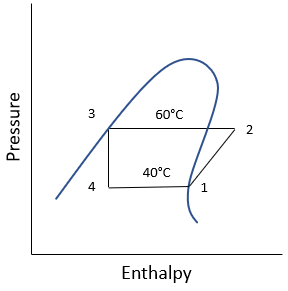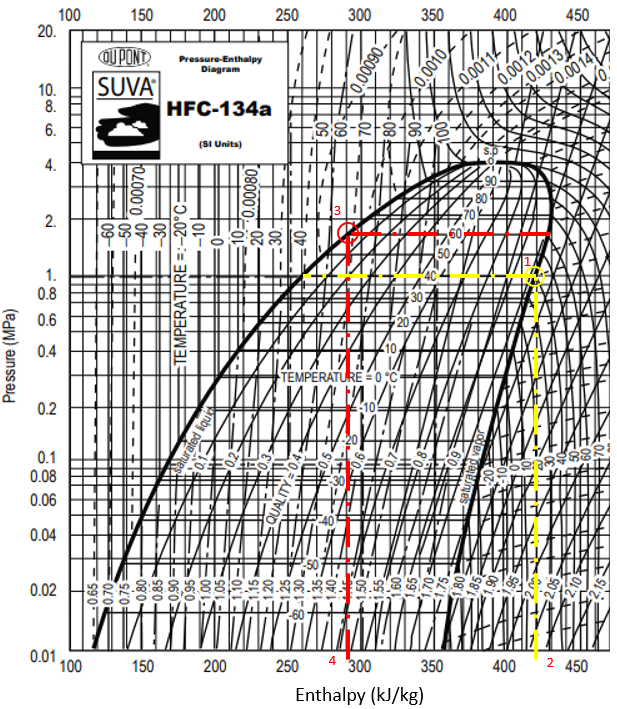## P-h Diagram

Refrigerant HFC-134a is used for a vapor-compression refrigeration cycle. If the evaporator temperature is 40°C, and the condenser temperature is 60°C, what is the evaporator cooling in kJ/kg?Hint
For this specific problem, the pressure vs enthalpy diagram is:Hint 2
For Boilers, Condensers, and Evaporators:
$$h_{in}+q=h_{exit}$$$where $$h$$ is specific enthalpy, and $$q$$ is the heat transfer per unit mass. For this specific problem, the pressure vs enthalpy diagram is:For Boilers, Condensers, and Evaporators: $$h_{in}+q=h_{exit}$$$
where $$h$$ is specific enthalpy, and $$q$$ is the heat transfer per unit mass. Per the problem statement’s P-h diagram:
$$q_{evaporator}=h_{1}-h_{4}$$$In the P-h diagram for Refrigerant HFC-134a, the enthalpy associated with the evaporator’s 40°C temperature intersecting with the saturated vapor curve (1) is $$h_1$$ (2). The enthalpy associated with condenser’s 60°C intersecting with the saturated liquid curve (3) is $$h_3$$ (4).Because $$h_3=h_4$$ based on the vapor-compression refrigeration cycle: $$q_{evaporator}=430\frac{kJ}{kg}-290\frac{kJ}{kg}=140\:\frac{kJ}{kg}$$$
140 kJ/kg